Courses

# Differentiator Amplifier - Applications of Op-amp, CSIR-NET Physical Sciences Physics Notes | EduRev

## Physics for IIT JAM, UGC - NET, CSIR NET

Created by: Akhilesh Thakur

## Physics : Differentiator Amplifier - Applications of Op-amp, CSIR-NET Physical Sciences Physics Notes | EduRev

The document Differentiator Amplifier - Applications of Op-amp, CSIR-NET Physical Sciences Physics Notes | EduRev is a part of the Physics Course Physics for IIT JAM, UGC - NET, CSIR NET.
All you need of Physics at this link: Physics

The basic operational amplifier differentiator circuit produces an output signal which is the first derivative of the input signal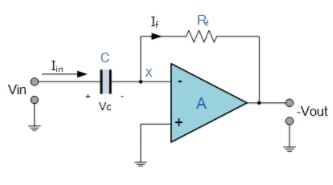Here, the position of the capacitor and resistor have been reversed and now the reactance, XC is connected to the input terminal of the inverting amplifier while the resistor, Rƒ forms the negative feedback element across the operational amplifier as normal.

This operational amplifier circuit performs the mathematical operation of Differentiation, that is it “produces a voltage output which is directly proportional to the input voltage’s rate-of-change with respect to time“. In other words the faster or larger the change to the input voltage signal, the greater the input current, the greater will be the output voltage change in response, becoming more of a “spike” in shape.

As with the integrator circuit, we have a resistor and capacitor forming an RC Networkacross the operational amplifier and the reactance ( Xc ) of the capacitor plays a major role in the performance of a Op-amp Differentiator.

Op-amp Differentiator Circuit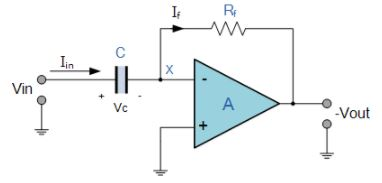The input signal to the differentiator is applied to the capacitor. The capacitor blocks any DC content so there is no current flow to the amplifier summing point, X resulting in zero output voltage. The capacitor only allows AC type input voltage changes to pass through and whose frequency is dependant on the rate of change of the input signal.

At low frequencies the reactance of the capacitor is “High” resulting in a low gain ( Rƒ/Xc ) and low output voltage from the op-amp. At higher frequencies the reactance of the capacitor is much lower resulting in a higher gain and higher output voltage from the differentiator amplifier.

However, at high frequencies an op-amp differentiator circuit becomes unstable and will start to oscillate. This is due mainly to the first-order effect, which determines the frequency response of the op-amp circuit causing a second-order response which, at high frequencies gives an output voltage far higher than what would be expected. To avoid this the high frequency gain of the circuit needs to be reduced by adding an additional small value capacitor across the feedback resistor Rƒ.

Since the node voltage of the operational amplifier at its inverting input terminal is zero, the current, i flowing through the capacitor will be given as: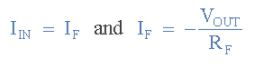The charge on the capacitor equals Capacitance times Voltage across the capacitor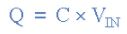Thus the rate of change of this charge is: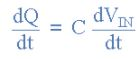but dQ/dt is the capacitor current, i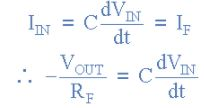from which we have an ideal voltage output for the op-amp differentiator is given as: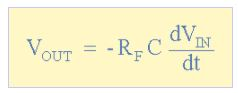Therefore, the output voltage Vout is a constant –Rƒ*C times the derivative of the input voltage Vin with respect to time. The minus sign (–) indicates a 180o phase shift because the input signal is connected to the inverting input terminal of the operational amplifier.

the Op-amp Differentiator circuit in its basic form has two main disadvantages compared to the previous operational amplifier integrator circuit. One is that it suffers from instability at high frequencies as mentioned above, and the other is that the capacitive input makes it very susceptible to random noise signals and any noise or harmonics present in the source circuit will be amplified more than the input signal itself. This is because the output is proportional to the slope of the input voltage so some means of limiting the bandwidth in order to achieve closed-loop stability is required.

Op-amp Differentiator Waveforms

If we apply a constantly changing signal such as a Square-wave, Triangular or Sine-wave type signal to the input of a differentiator amplifier circuit the resultant output signal will be changed and whose final shape is dependant upon the RC time constant of the Resistor/Capacitor combination.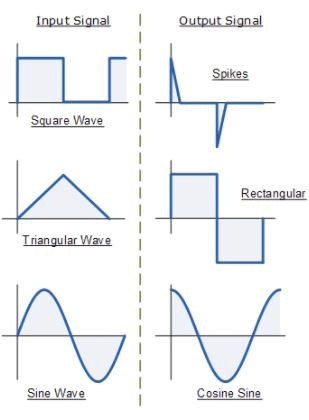Improved Op-amp Differentiator Amplifier

The basic single resistor and single capacitor op-amp differentiator circuit is not widely used to reform the mathematical function of Differentiation because of the two inherent faults mentioned above, “Instability” and “Noise”. So in order to reduce the overall closed-loop gain of the circuit at high frequencies, an extra resistor, Rin is added to the input as shown below.

Improved Op-amp Differentiator Amplifier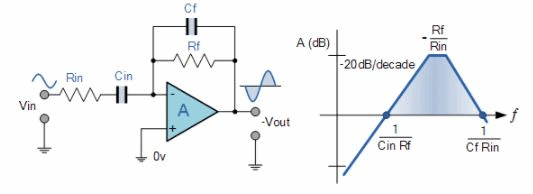Adding the input resistor RIN limits the differentiators increase in gain at a ratio of Rƒ/RINThe circuit now acts like a differentiator amplifier at low frequencies and an amplifier with resistive feedback at high frequencies giving much better noise rejection.

Additional attenuation of higher frequencies is accomplished by connecting a capacitor Cƒ in parallel with the differentiator feedback resistor, Rƒ. This then forms the basis of a Active High Pass Filter as we have seen before in the filters section.

Offer running on EduRev: Apply code STAYHOME200 to get INR 200 off on our premium plan EduRev Infinity!

159 docs

,

,

,

,

,

,

,

,

,

,

,

,

,

,

,

,

,

,

,

,

,

,

,

,

;